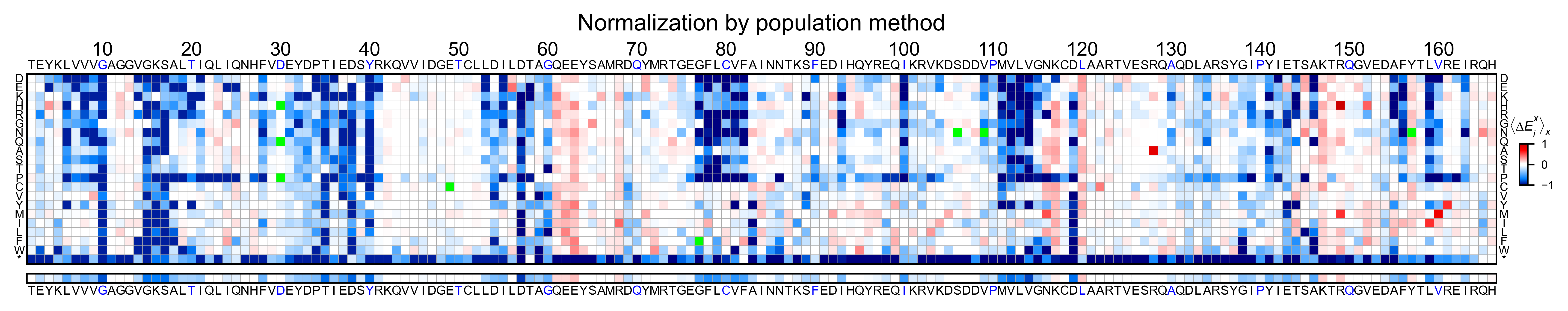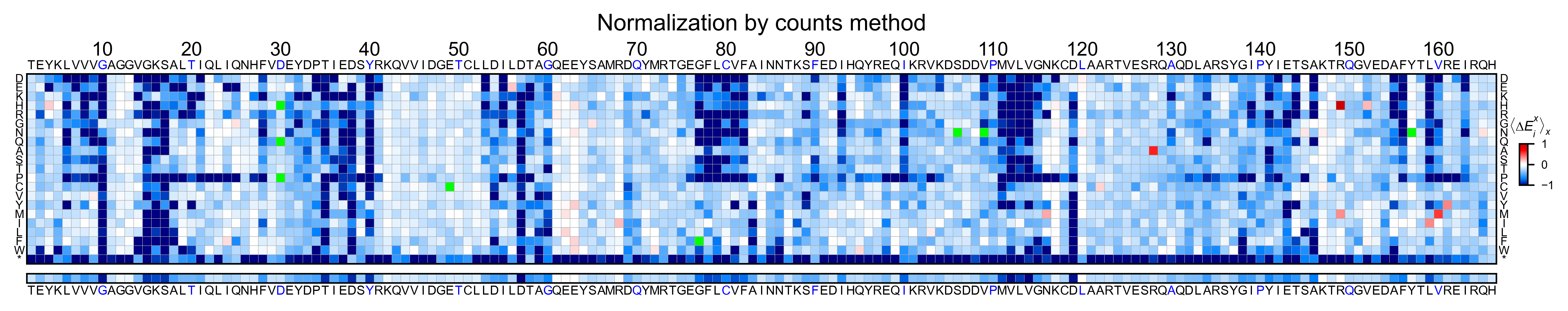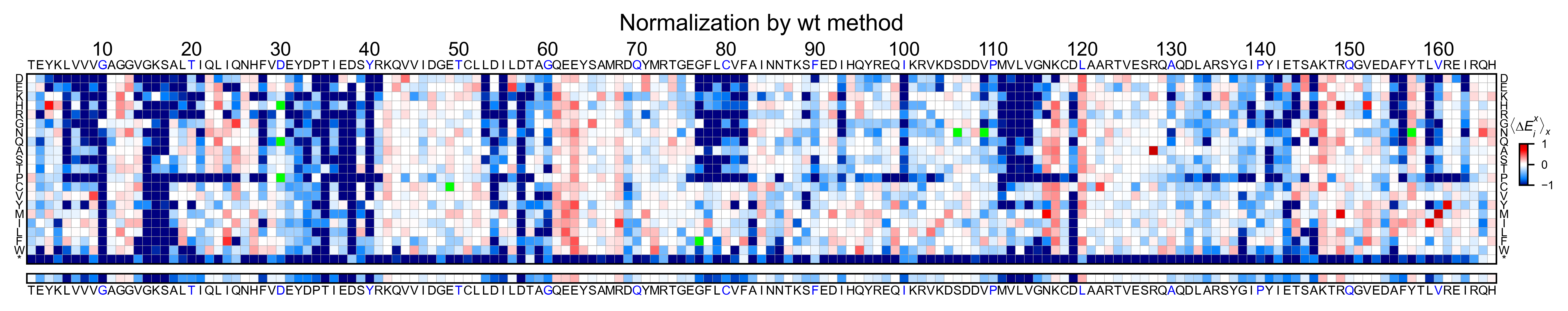# Normalizing datasets¶

This section will teach the different options to normalize the data using the function `mutagenesis_visualization.calculate_enrichment()` .

If you already have your own processing pipeline built, you can skip this section and go to the (Creating plots) examples.

## Import modules and load data¶

```%matplotlib inline
from typing import List, Dict
import numpy as np
import pandas as pd
from pandas.core.frame import DataFrame

from mutagenesis_visualization import calculate_enrichment
from mutagenesis_visualization.main.utils.data_paths import HRAS_RBD_COUNTS
from mutagenesis_visualization import Screen
```

```# Define protein sequence
hras_sequence: str = 'MTEYKLVVVGAGGVGKSALTIQLIQNHFVDEYDPTIEDSYRKQVVIDGETCLLDILDTAGQEEY'\
+ 'SAMRDQYMRTGEGFLCVFAINNTKSFEDIHQYREQIKRVKDSDDVPMVLVGNKCDLAARTVES'\
+ 'RQAQDLARSYGIPYIETSAKTRQGVEDAFYTLVREIRQHKLRKLNPPDESGPG'

# First residue of the hras_enrichment dataset. Because 1-Met was not mutated, the dataset starts at residue 2
start_position: int = 2

# Substitute Nan values with 0
fillna: int = 0
```
```# List of sheets and columns to use
sheets_pre: List[str] = ['R1_before', 'R2_before', 'R3_before']
sheets_sel: List[str] = ['R1_after', 'R2_after', 'R3_after']
columns: List[str] = ['F:BG', 'BH:DK', 'DL:FN']
columns_wt: List[str] = ['A', 'B', 'C']

# Create dictionary with data. Loading 3 replicates, each of them is divided into 3 pools
dict_pre, dict_sel, dict_pre_wt, dict_sel_wt = ({} for i in range(4))

# Read counts from file (could be txt, csv, xlsx, etc...)
for column, column_wt in zip(columns, columns_wt):
for sheet_pre, sheet_sel in zip(sheets_pre, sheets_sel):
# Pre counts
label_pre = str(sheet_pre + '_' + column_wt)
HRAS_RBD_COUNTS, sheet_pre, skiprows=1, usecols=column, nrows=32
)
# Pre counts wild-type alleles
HRAS_RBD_COUNTS, sheet_pre, usecols=column_wt
)

# Sel counts
label_sel = str(sheet_sel + '_' + column_wt)
HRAS_RBD_COUNTS, sheet_sel, skiprows=1, usecols=column, nrows=32
)
# Sel counts wild-type alleles
HRAS_RBD_COUNTS, sheet_sel, usecols=column_wt
)
```

## Calculate log10 enrichment¶

Now we are going to calculate the log10(sel/pre) for the sublibrary 1 of each replicate and plot a histogram. The resulting distribution is bimodal, and because the three replicates have a similar number of counts ratios, their center is overlapping. However, because we have not normalized by the number of counts, and there are more counts in the selected than in the pre-selected population, the center is >0.

```# Auxiliar function to convert +-inf values to an arbitrary number (ie +-2)
def _replace_inf(df: DataFrame) -> DataFrame:
df.replace(to_replace=np.inf, value=2, inplace=True)
df.replace(to_replace=-np.inf, value=-2, inplace=True)
return df

enrichment = {}

# calculate log10 enrichment for each replicate
for pre_key, sel_key in zip(list(dict_pre.keys())[:3],
list(dict_sel.keys())[:3]):
# log 10
enrichment_log10 = (np.log10(dict_sel[sel_key] / dict_pre[pre_key]))
enrichment_log10['aminoacids'] = aminoacids
enrichment_log10.set_index(['aminoacids'], inplace=True)
enrichment[pre_key[:2]] = _replace_inf(enrichment_log10)

# Create objects
hras_object: Screen = Screen(
list(enrichment.values()), hras_sequence, aminoacids, start_position, fillna,
)

hras_object.kernel(show_replicates=True, kernel_color_replicates = ["b", "r", "g"], title=r'\$log_{10}\$' + '(sel/pre)', xscale=(-1, 1))
```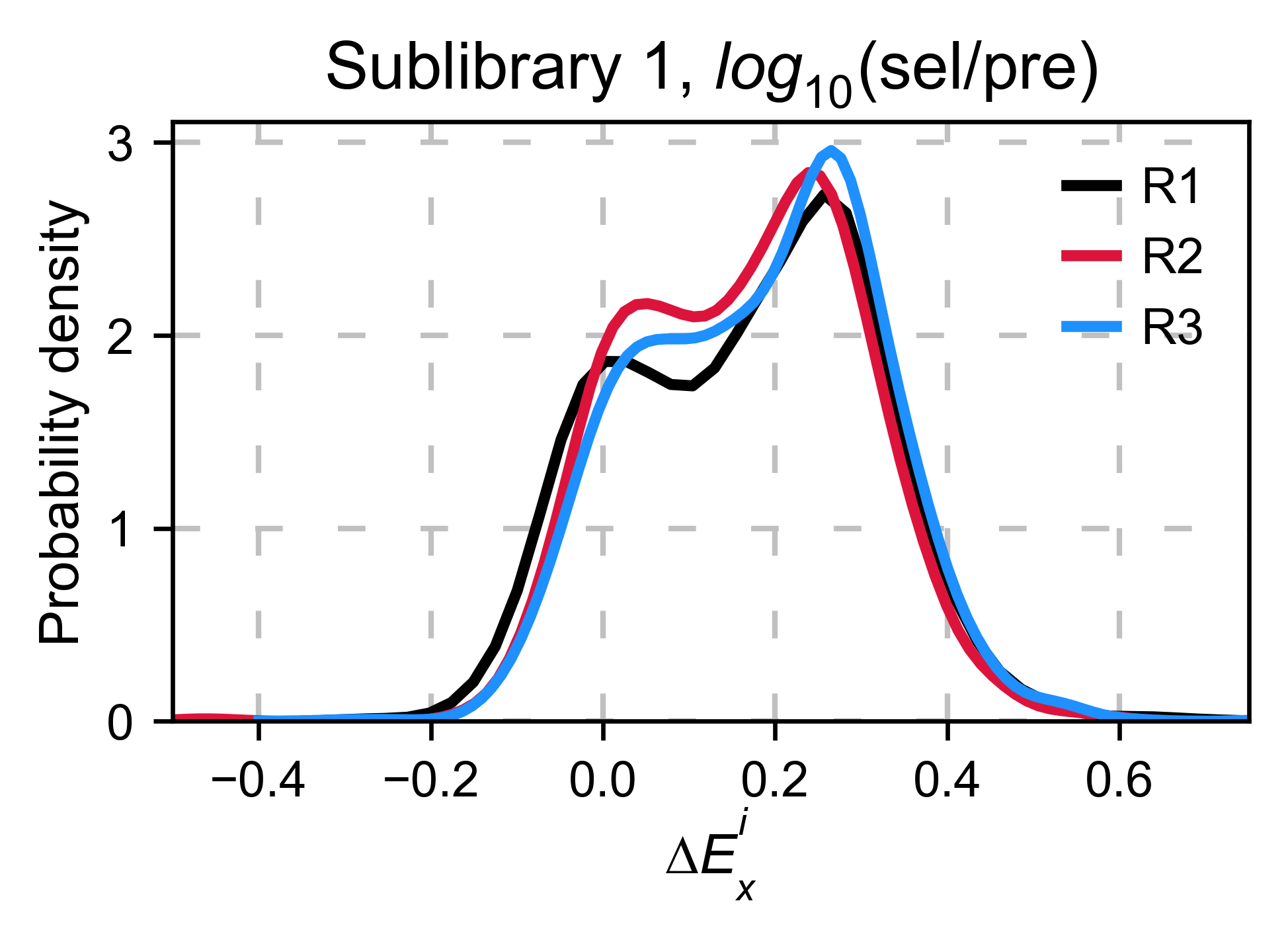## Centering the data (zeroing)¶

Functions used in this section:

### Counts normalization¶

Normalizing by the number of counts improves normalization. Now the population center is closer to 0. To do so, set `zeroing_method='counts'`.

```enrichment = {}
# calculate log10 enrichment for each replicate
for pre_key, sel_key in zip(list(dict_pre.keys())[:3],
list(dict_sel.keys())[:3]):
# Enrichment
enrichment[pre_key[:2]] = calculate_enrichment(
aminoacids, dict_pre[pre_key], dict_sel[sel_key], zeroing_method='counts', stopcodon=False
)

# Plot histogram and KDE
aminoacids: List[str] = list('ACDEFGHIKLMNPQRSTVWY*')

hras_object: Screen = Screen(
list(enrichment.values()), hras_sequence, aminoacids, start_position, fillna,
)

hras_object.kernel(show_replicates=True, kernel_color_replicates = ["b", "r", "g"], title='zeroing_method = counts', xscale=(-1, 0.5))
```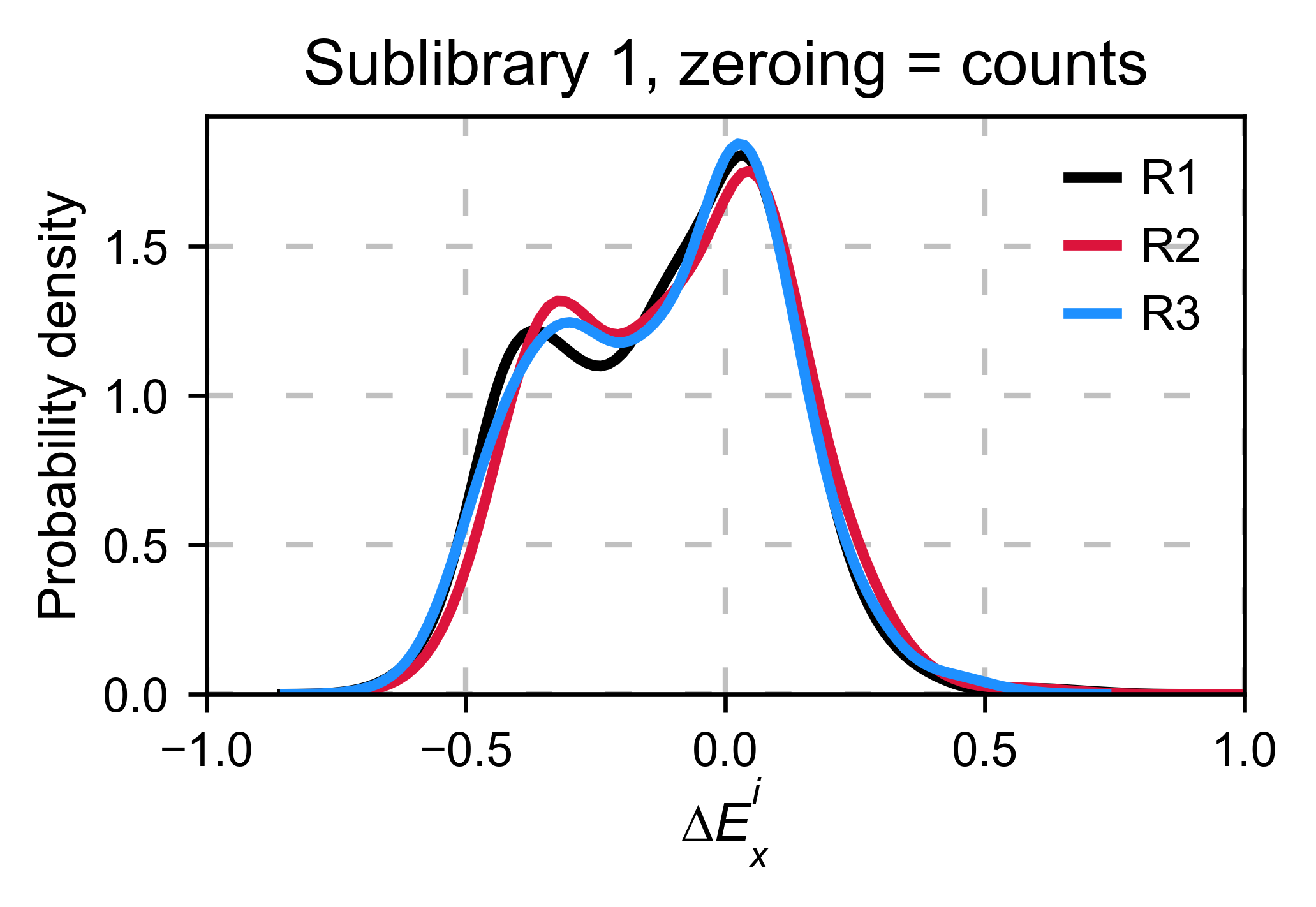### Wt allele¶

Another way we can normalize is by using an internal reference such as a particular mutant. In the following example we will use the wild-type allele. If the assay that you are using is noisy, relying on a single data point for normalizing will result in high variance. The package does not include this option because it may lead to errors. Here we are showing how it would be done by hand. In this example, it works fine. But in other datasets we have, it has been a source of error.

```# calculate log10 enrichment for each replicate

enrichment = {}

# calculate log10 enrichment for each replicate
for pre_key, sel_key in zip(list(dict_pre.keys())[:3],
list(dict_sel.keys())[:3]):
# log 10
wt_ratio = np.log10(
dict_sel_wt[sel_key]['wt 2-56'] / dict_pre_wt[pre_key]['wt 2-56']
)
enrichment_log10 = np.log10(
dict_sel[sel_key] / dict_pre[pre_key]
) - wt_ratio
enrichment_log10['aminoacids'] = aminoacids
enrichment_log10.set_index(['aminoacids'], inplace=True)
enrichment[pre_key[:2]] = _replace_inf(enrichment_log10)

hras_object: Screen = Screen(
list(enrichment.values()), hras_sequence, aminoacids, start_position, fillna,
)
hras_object.kernel(show_replicates=True, kernel_color_replicates = ["b", "r", "g"], title='zeroing_method = wt allele only', xscale=(-0.5, 0.5))
```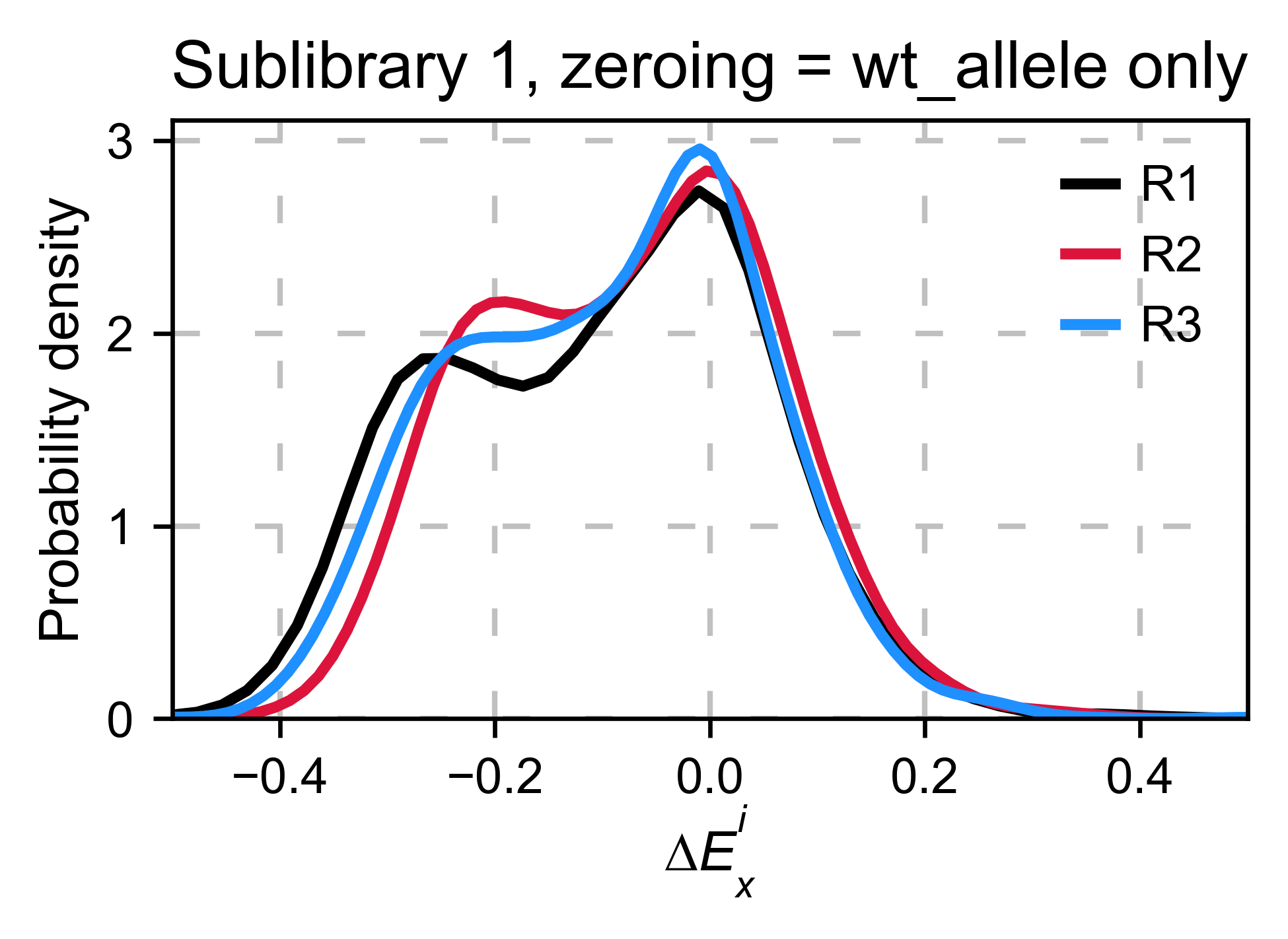### Distribution of synonymous wt alleles¶

In our experience, it is better to use the median/mode/mean of the synonymous wild-type population because there is less variance. `calculate_enrichment` has such an options by using `zeroing_method='wt'` and then `zeroing_metric ='median', 'mean' or 'mode'`.

```enrichment = {}

# calculate log10 enrichment for each replicate
for pre_key, sel_key in zip(list(dict_pre.keys())[:3],
list(dict_sel.keys())[:3]):
# Enrichment
enrichment[pre_key[:2]] = calculate_enrichment(
aminoacids,
dict_pre[pre_key],
dict_sel[sel_key],
dict_pre_wt[pre_key],
dict_sel_wt[sel_key],
zeroing_method='wt',
zeroing_metric ='mode',
stopcodon=False
)

aminoacids: List[str] = list('ACDEFGHIKLMNPQRSTVWY*')

hras_object: Screen = Screen(
list(enrichment.values()), hras_sequence, aminoacids, start_position, fillna,
)
hras_object.kernel(show_replicates=True, title='Sublibrary 1, zeroing_method = wt', xscale=(-1.5, 1))
```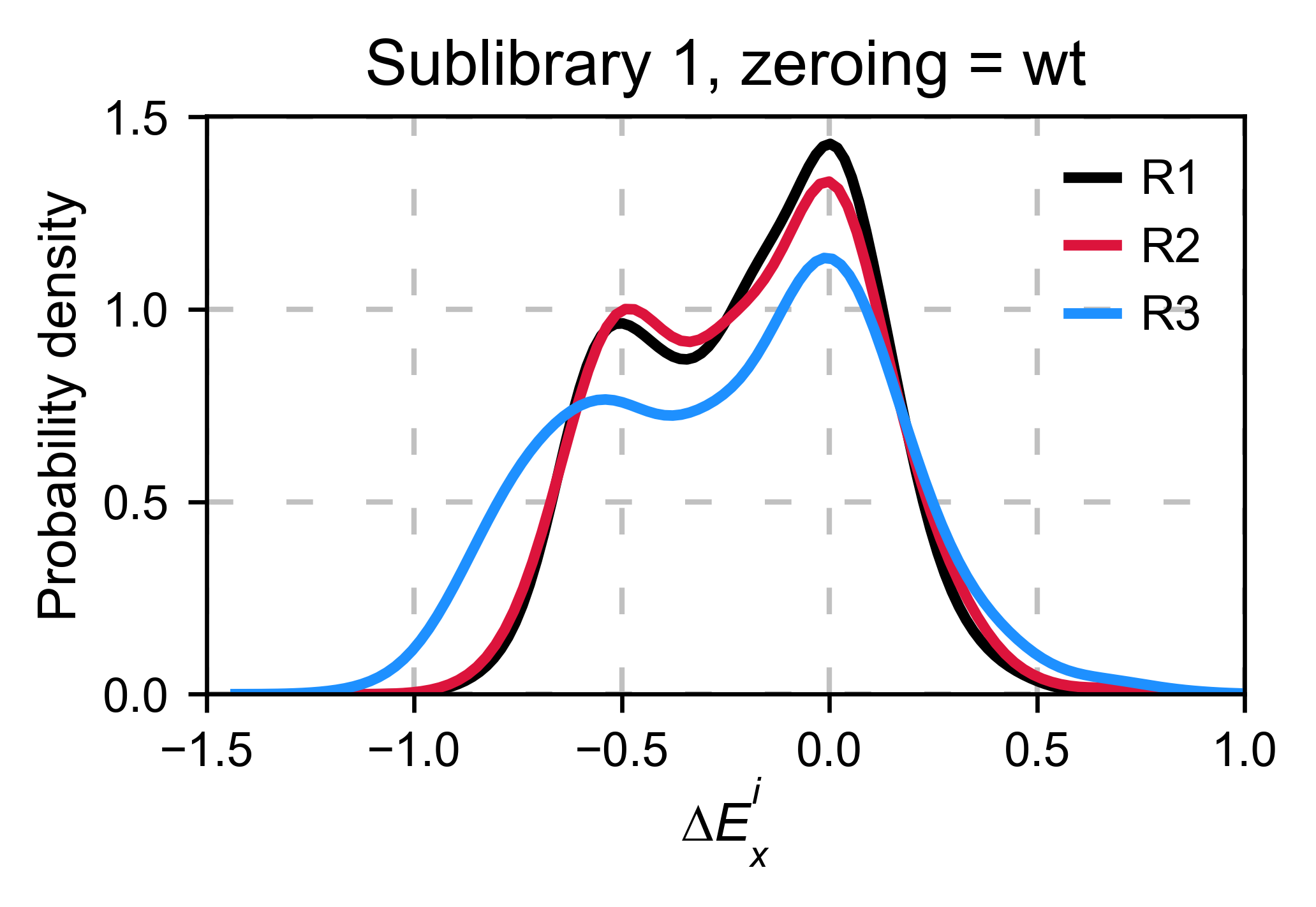### Wt alleles observation¶

If the population of synonymous wild-type alleles (alleles that are wild-type at a protein level, but not at a DNA level) is small, the distribution of this variants may have high variance from sample to sample. Also, you will notice that not all wild-type alleles are neutral. The spread of these alleles gives a sense of the noise in the experiment.

At least for the following data, there is no correlation between the performance of wild-type alleles in different replicates, suggesting that the higher or lower enrichment scores are caused by noise and not a fitness difference caused by changes in protein expression.

```hras_object.kernel(show_replicates=True, wt_counts_only=True,title='Wild-type alleles', kernel_colors=['k', 'crimson', 'dodgerblue', 'g', 'silver'], xscale=(-0.5, 1), output_file="docs/images/exported_images/hras_wildtype_distribution.png")
```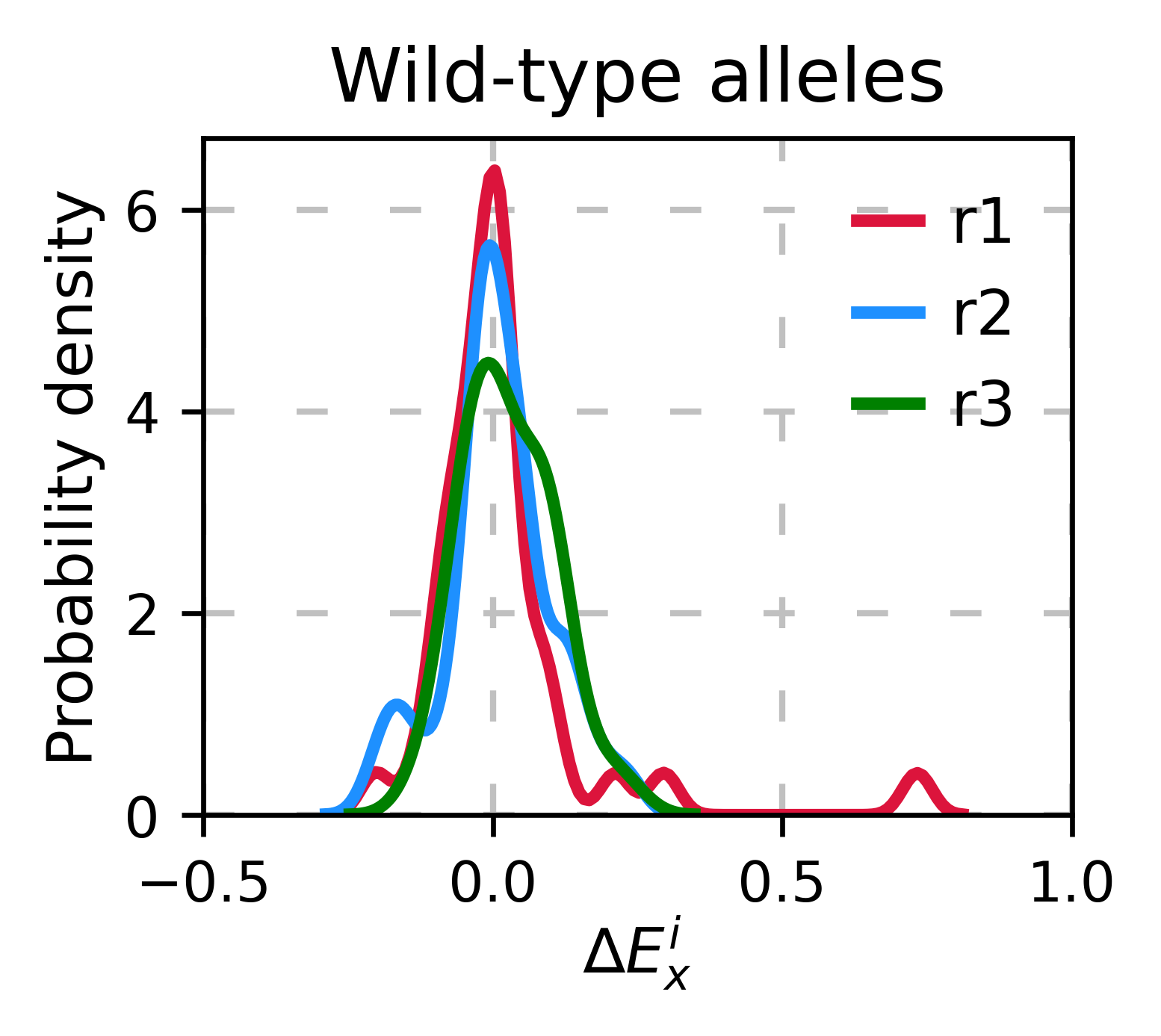Perform the scatter plots:

```hras_object.scatter_replicates(wt_counts_only=True,title='Wild-type alleles', xscale=(-1, 1), yscale=(-1, 1), output_file="docs/images/exported_images/hras_wildtype_scatter.png")
```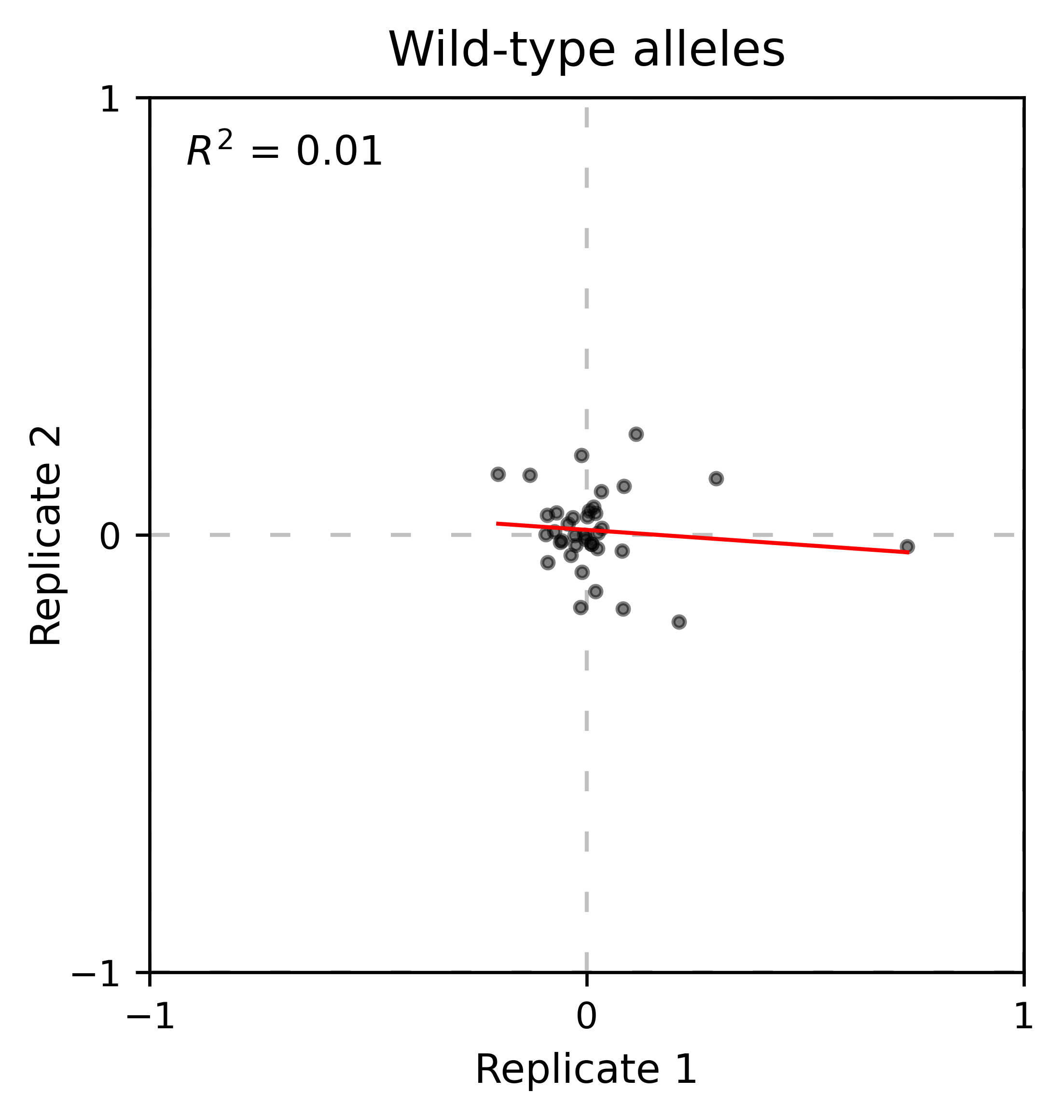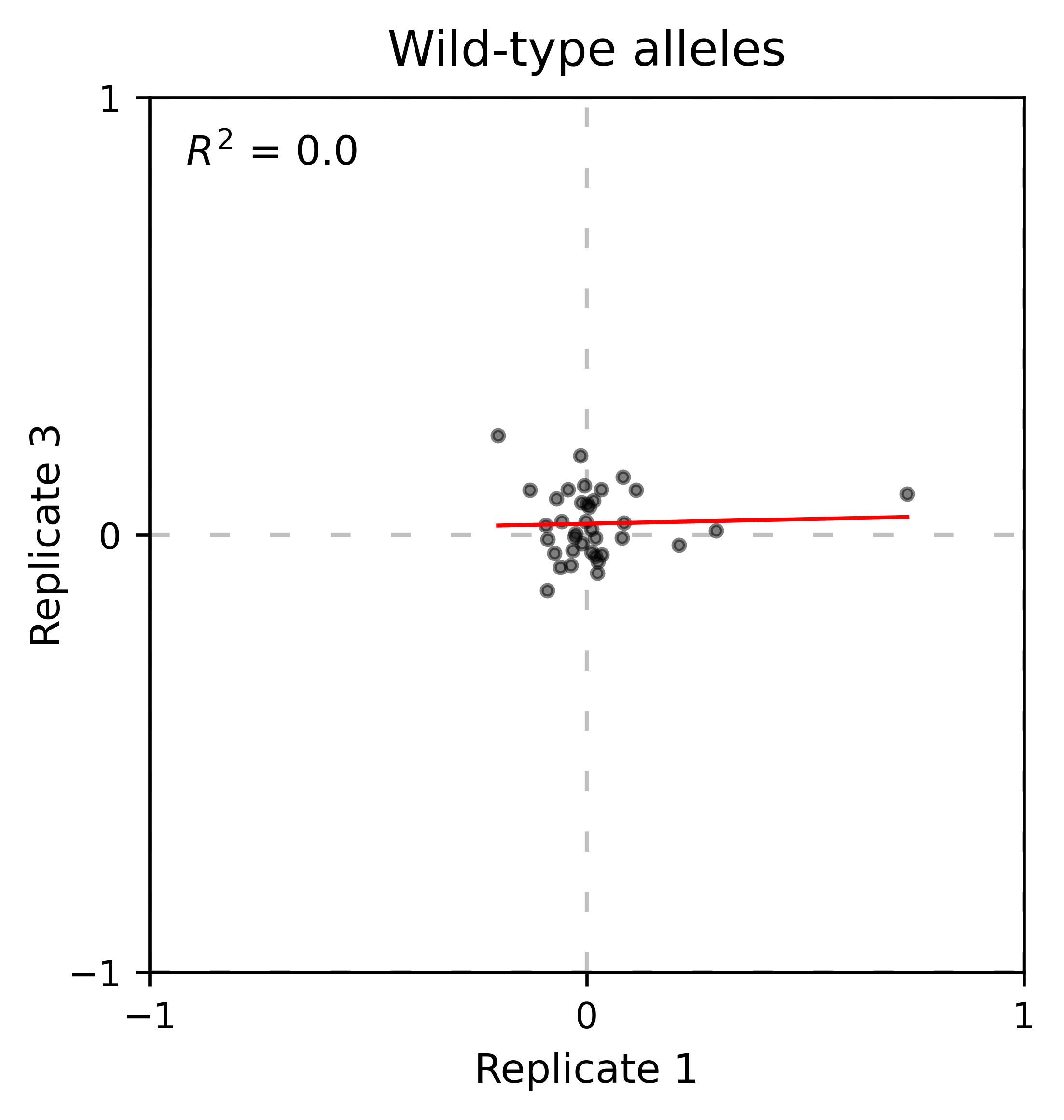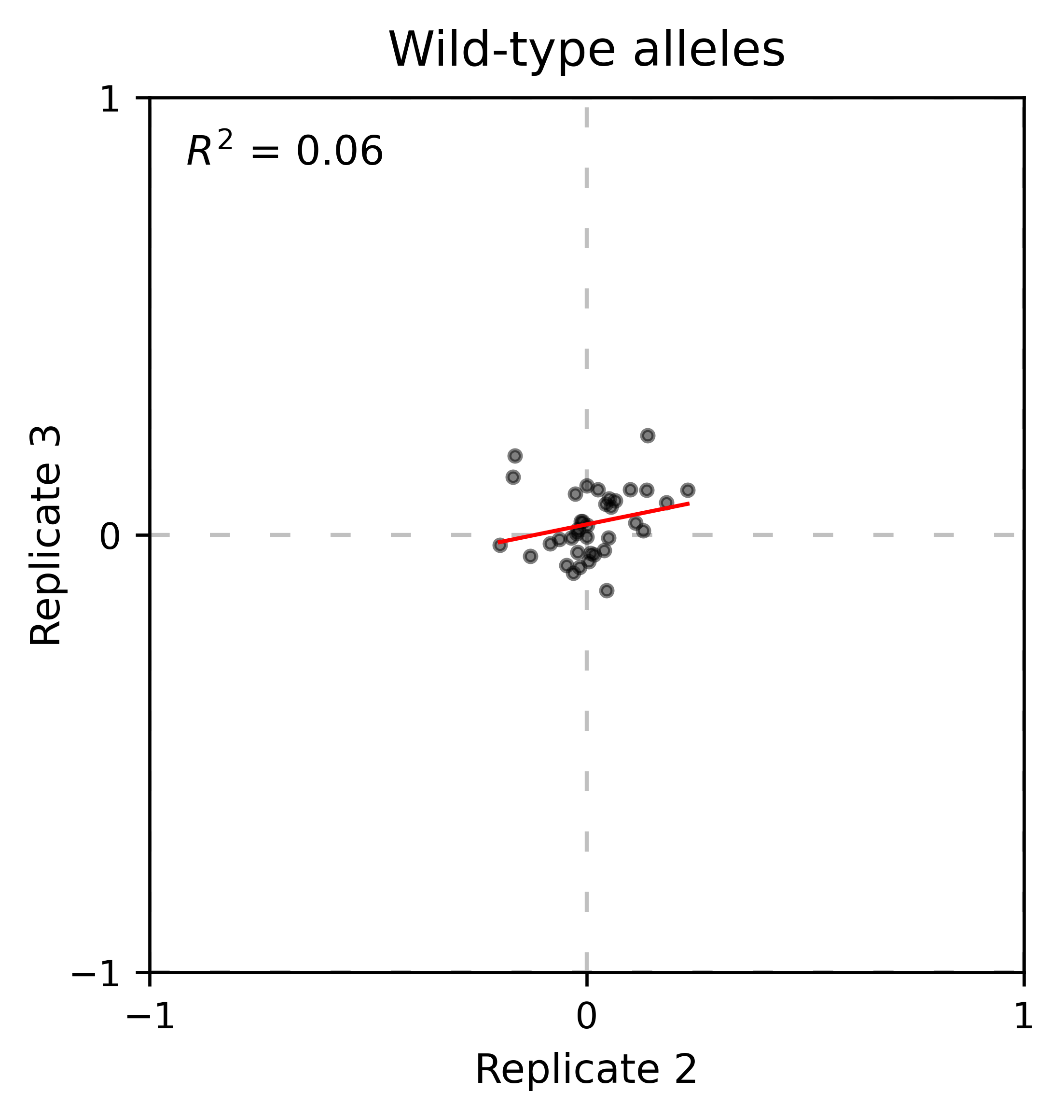### Distribution of mutants¶

An alternative option to normalize the data is to use the mean/median/mode of the population to some specific number such as zero. To do so, use `zeroing_method='population'`. The parameters of the distribution will be calculated assuming a gaussian distribution. Not only the three replicates are centered, but also they have the same spread.

```enrichment = {}

# calculate log10 enrichment for each replicate
for pre_key, sel_key in zip(list(dict_pre.keys())[:3],
list(dict_sel.keys())[:3]):
# Enrichment
enrichment[pre_key[:2]]  = calculate_enrichment(
aminoacids,
dict_pre[pre_key],
dict_sel[sel_key],
zeroing_method='population',
zeroing_metric ='mode',
stopcodon=False
)

aminoacids: List[str] = list('ACDEFGHIKLMNPQRSTVWY*')

hras_object: Screen = Screen(
list(enrichment.values()), hras_sequence, aminoacids, start_position, fillna,
)
hras_object.kernel(show_replicates=True, title='zeroing_method = population', xscale=(-1, 1))
```A variant of the previous method is to calculate the kernel density estimate using `zeroing_method='kernel'`. This option centers the population using the mode of the KDE. If the data is bimodal, it will select the main peak. Furthermore, it will use the standard deviation of the main peak to scale the data. This method is useful when you have split your library into multiple pools because it will not only center the data properly but also do scale the data so each pool main peak has the same standard deviation. Results are quite similar to setting `zeroing_method='population'` and `zeroing_metric ='mode'`.

```enrichment = {}

# calculate log10 enrichment for each replicate
for pre_key, sel_key in zip(list(dict_pre.keys())[:3],
list(dict_sel.keys())[:3]):
# Enrichment
enrichment[pre_key[:2]] = calculate_enrichment(
aminoacids, dict_pre[pre_key], dict_sel[sel_key], zeroing_method='kernel', stopcodon=False
)

aminoacids: List[str] = list('ACDEFGHIKLMNPQRSTVWY*')

hras_object: Screen = Screen(
list(enrichment.values()), hras_sequence, aminoacids, start_position, fillna,
)
hras_object.kernel(show_replicates=True, kernel_color_replicates = ["b", "r", "g"],  title='zeroing method = kernel', xscale=(-1.5,1))
```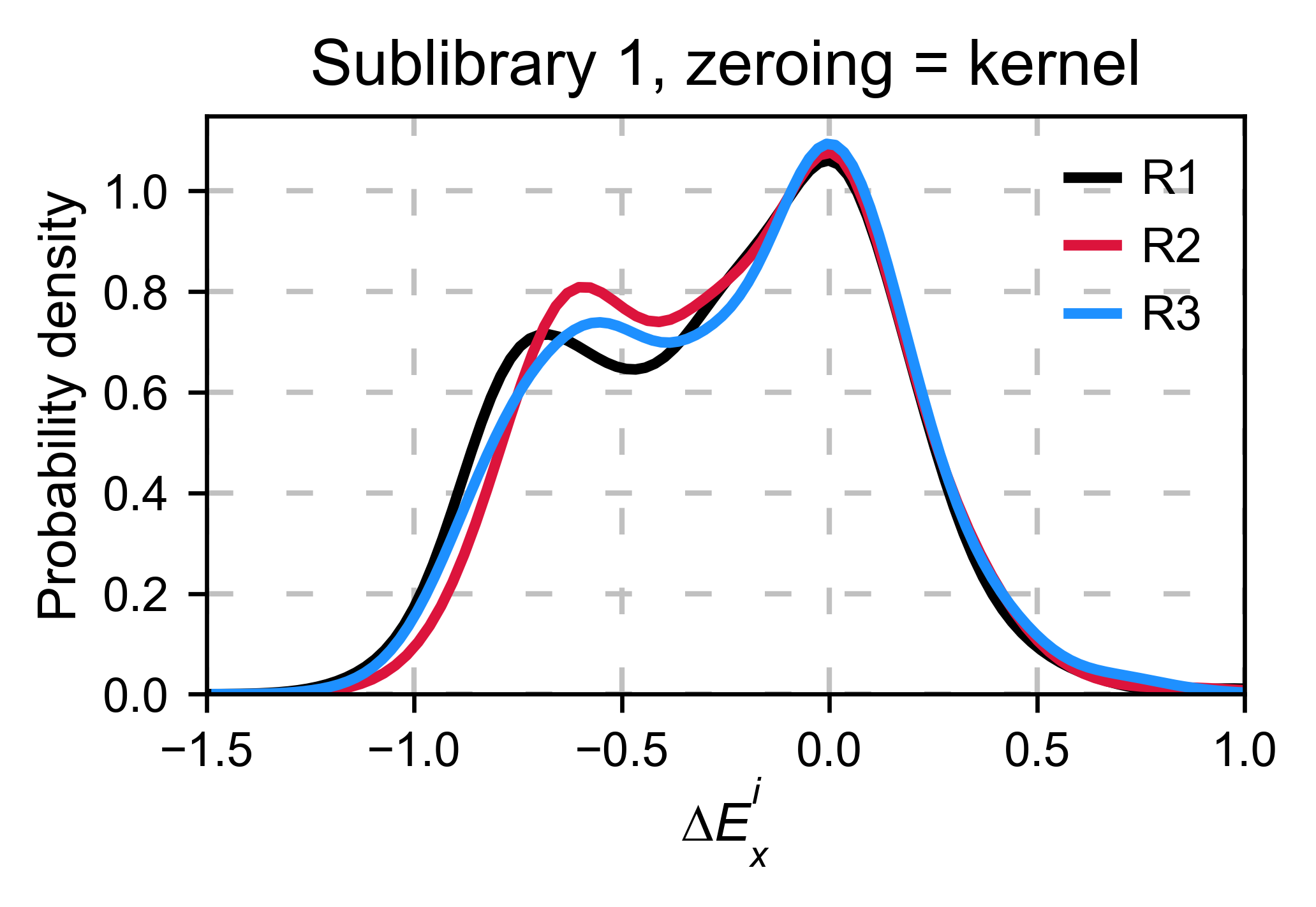## Baseline subtraction¶

Including stop codons in the library can be of great use because it gives a control for basal signal in your assay. The algorithm has the option to apply a baseline subtraction. The way it works is it sets the stop codons counts of the selected population to 0 (baseline) and subtracts the the baseline signal to every other mutant. To use this option, set `stopcodon=True`. You will notice that it get rids of the shoulder peak, and now the distribution looks unimodal with a big left shoulder.

```enrichment = {}

# calculate log10 enrichment for each replicate
for pre_key, sel_key in zip(list(dict_pre.keys())[:3],
list(dict_sel.keys())[:3]):
# Enrichment
enrichment[pre_key[:2]] = calculate_enrichment(
aminoacids, dict_pre[pre_key], dict_sel[sel_key], zeroing_method='kernel', stopcodon=True
)

aminoacids: List[str] = list('ACDEFGHIKLMNPQRSTVWY*')

hras_object: Screen = Screen(
list(enrichment.values()), hras_sequence, aminoacids, start_position, fillna,
)
hras_object.kernel(show_replicates=True, kernel_color_replicates = ["b", "r", "g"], title='stop codon correction', xscale=(-5, 1.5))
```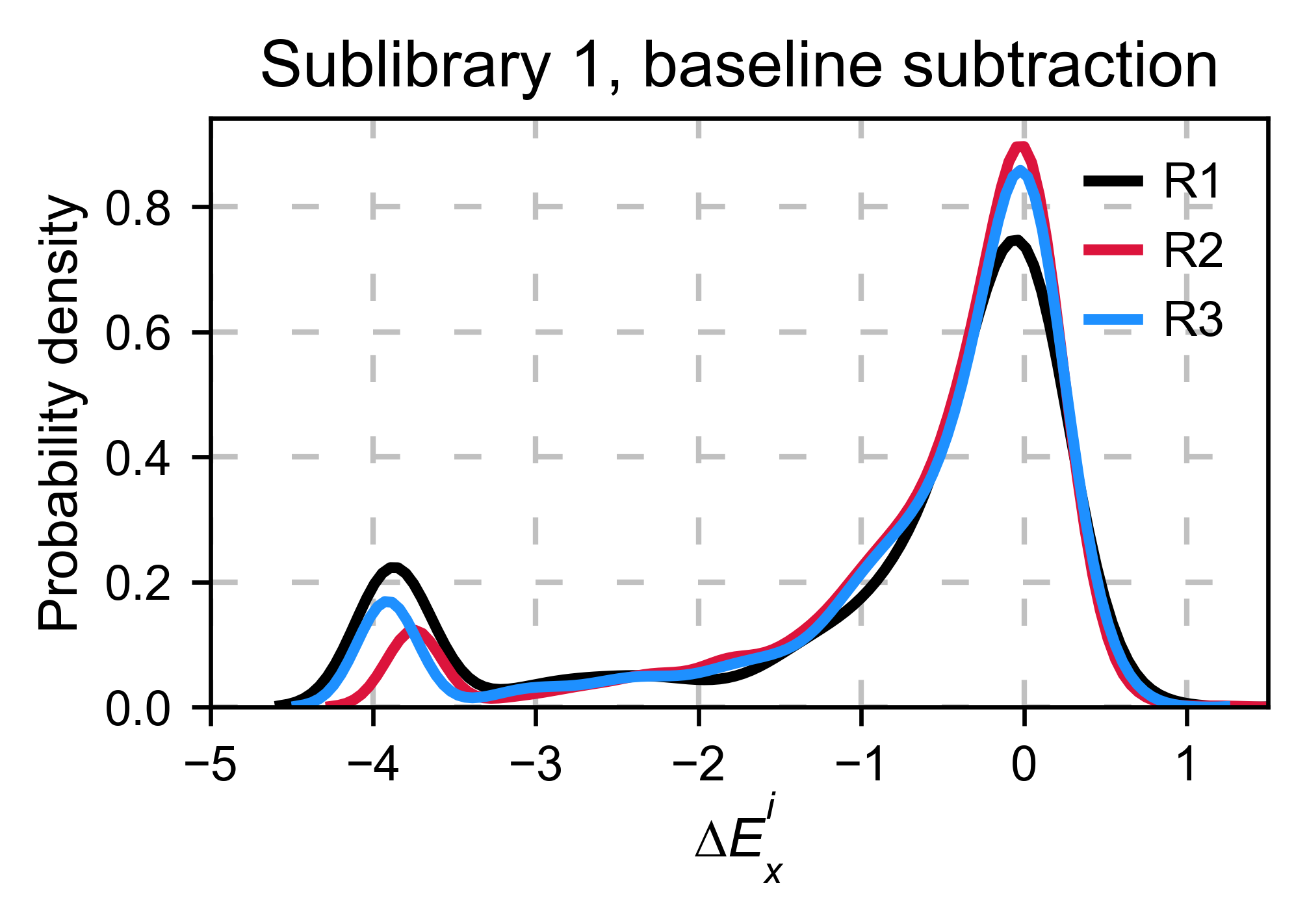## Scaling¶

By now you probably have realized that different options of normalization affect to the spread of the data. The rank between each mutant is unchanged between the different methods, so it is a matter of multiplying/dividing by a scalar to adjust the data spread. Changing the value of the parameter `std_scale` will do the job. You will probably do some trial an error until you find the right value. In the following example we are changing the `std_scale` parameter for each of the three replicates shown. Note that the higher the scalar, the higher the spread.

```enrichment_scalar = {}
scalars: List[str] = [0.1, 0.2, 0.3]

# calculate log10 enrichment for each replicate
for pre_key, sel_key, scalar in zip(list(dict_pre.keys())[:3],
list(dict_sel.keys())[:3], scalars):
# Enrichment
enrichment_log10 = calculate_enrichment(
aminoacids,
dict_pre[pre_key],
dict_sel[sel_key],
zeroing_method='kernel',
stopcodon=True,
std_scale=scalar
)
enrichment_scalar[pre_key[:2]] = enrichment_log10

aminoacids: List[str] = list('ACDEFGHIKLMNPQRSTVWY*')

hras_object: Screen = Screen(
list(enrichment_scalar.values()), hras_sequence, aminoacids, start_position, fillna,
)
hras_object.kernel(show_replicates=True, kernel_color_replicates = ["b", "r", "g"], title='scaling', xscale=(-5, 1.5))
```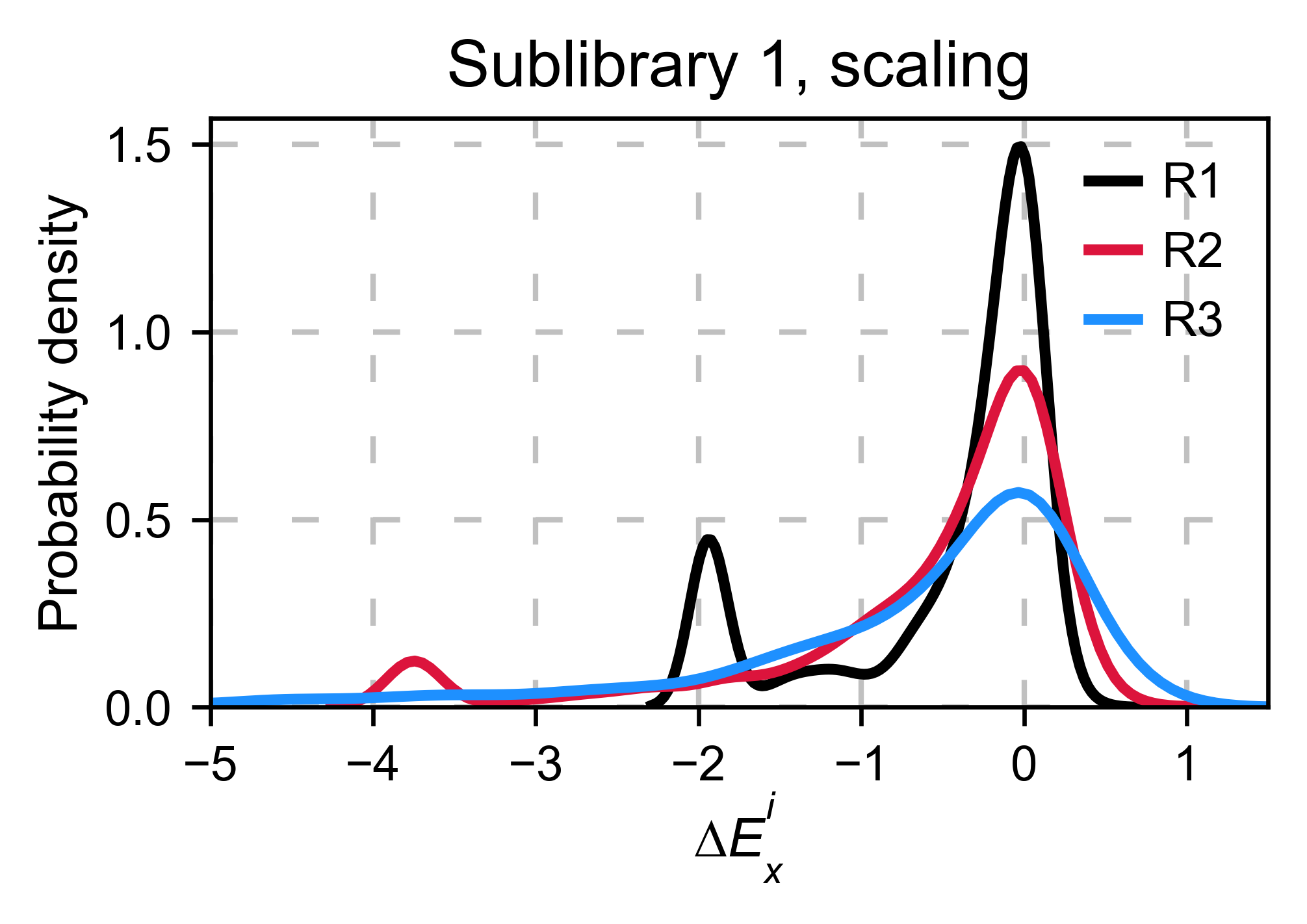## Multiple sublibraries¶

In our own research projects, where we have multiple DNA pools, we have determined that the combination of parameters that best suit us it to the wild-type synonymous sequences to do a first data normalization step. Then use `zeroing_method = 'kernel'` to zero the data and use `stopcodon=True` in order to determine the baseline level of signal. You may need to use different parameters for your purposes. Feel free to get in touch if you have questions regarding data normalization.

```# Labels
labels: List[str] = ['Sublibrary 1', 'Sublibrary 2', 'Sublibrary 3']
zeroing_options: List[str] = ['population', 'counts', 'wt', 'kernel']
title: str = 'Rep-A sublibraries, zeroing_method = '

# xscale
xscales = [(-2, 1), (-2.5, 0.5), (-3.5, 1.5), (-3.5, 1.5)]
# declare dictionary
enrichment_lib = {}
df_lib = {}

for option, xscale in zip(zeroing_options, xscales):
for pre_key, sel_key, label in zip(list(dict_pre.keys())[::3],
list(dict_sel.keys())[::3], labels):

# log 10
enrichment_lib[label]  = DataFrame(calculate_enrichment(
aminoacids,
dict_pre[pre_key],
dict_sel[sel_key],
dict_pre_wt[pre_key],
dict_sel_wt[sel_key],
zeroing_method=option,
zeroing_metric ='mode',
stopcodon=True,
infinite=2
))

# Concatenate sublibraries and store in dict
df_lib[option] = pd.concat([
enrichment_lib['Sublibrary 1'], enrichment_lib['Sublibrary 2'],
enrichment_lib['Sublibrary 3']
],ignore_index=True, axis=1)

# Plot
aminoacids: List[str] = list('ACDEFGHIKLMNPQRSTVWY*')

hras_sublibrary1: Screen = Screen(
enrichment_lib['Sublibrary 1'], hras_sequence, aminoacids, start_position, fillna,
)
hras_sublibrary2: Screen = Screen(
enrichment_lib['Sublibrary 2'], hras_sequence, aminoacids, start_position, fillna,
)
hras_sublibrary3: Screen = Screen(
enrichment_lib['Sublibrary 3'], hras_sequence, aminoacids, start_position, fillna,
)
hras_sublibrary1.multiple_kernel([hras_sublibrary2, hras_sublibrary3], label_kernels = labels, title=title + option, xscale=xscale)
```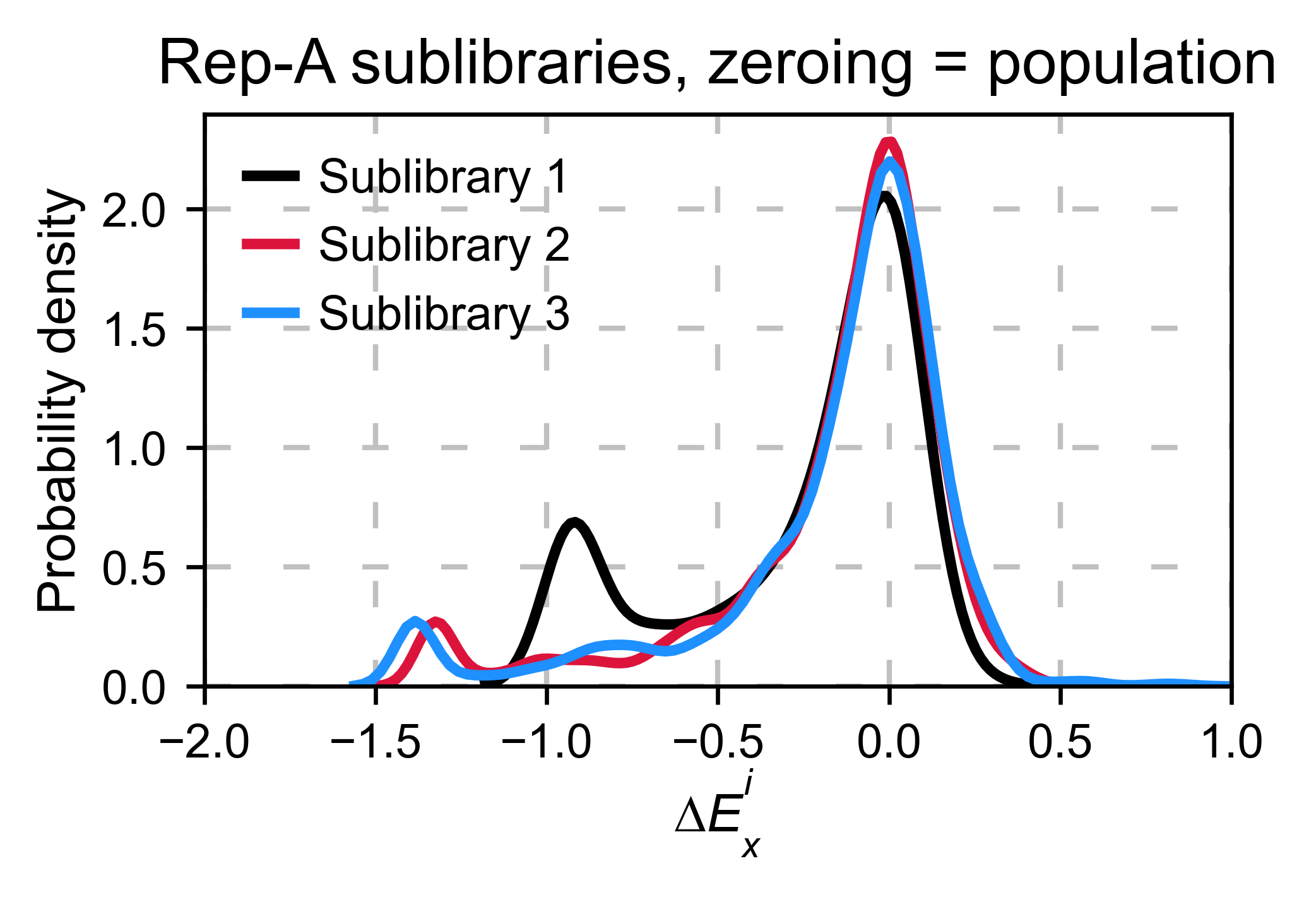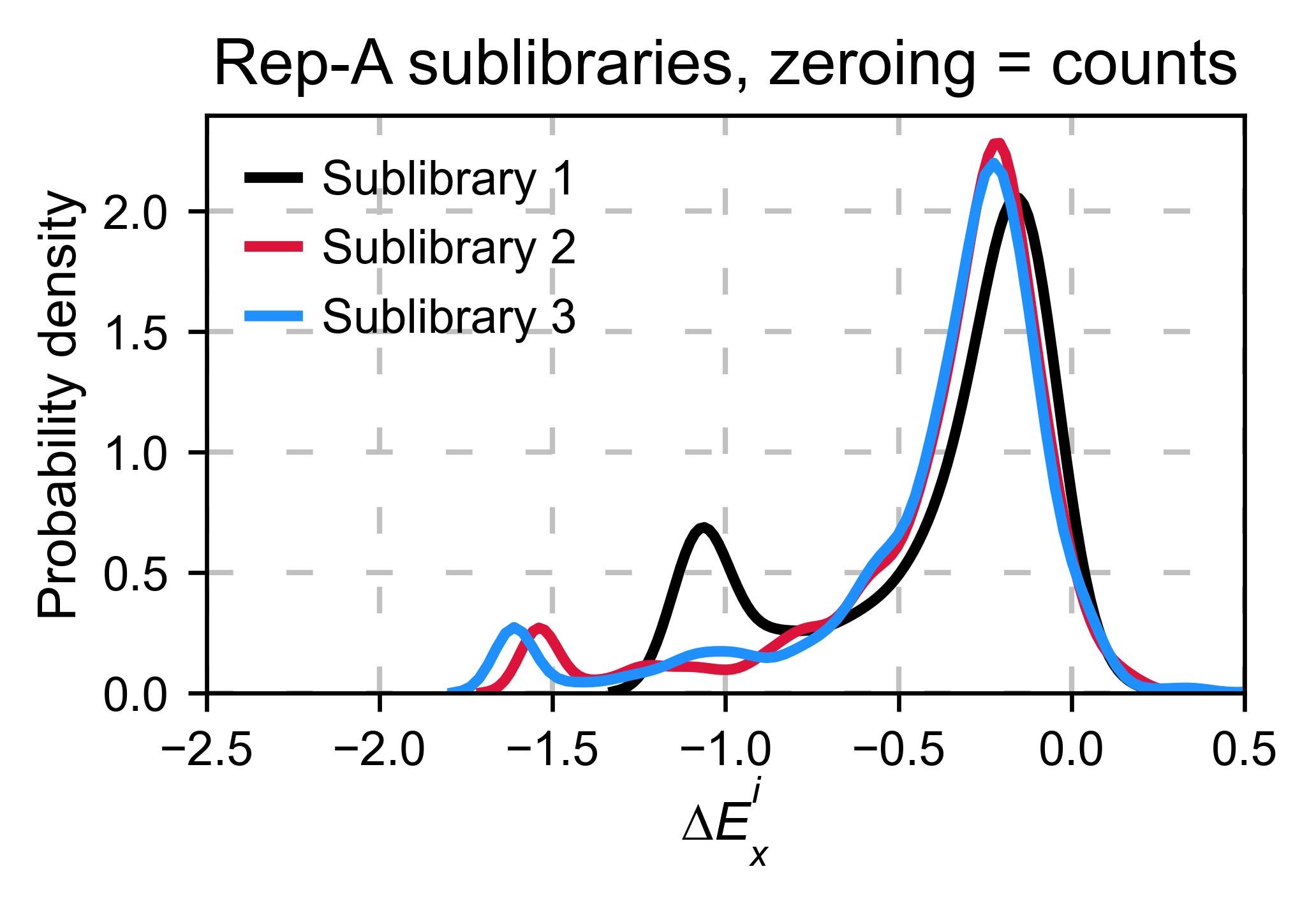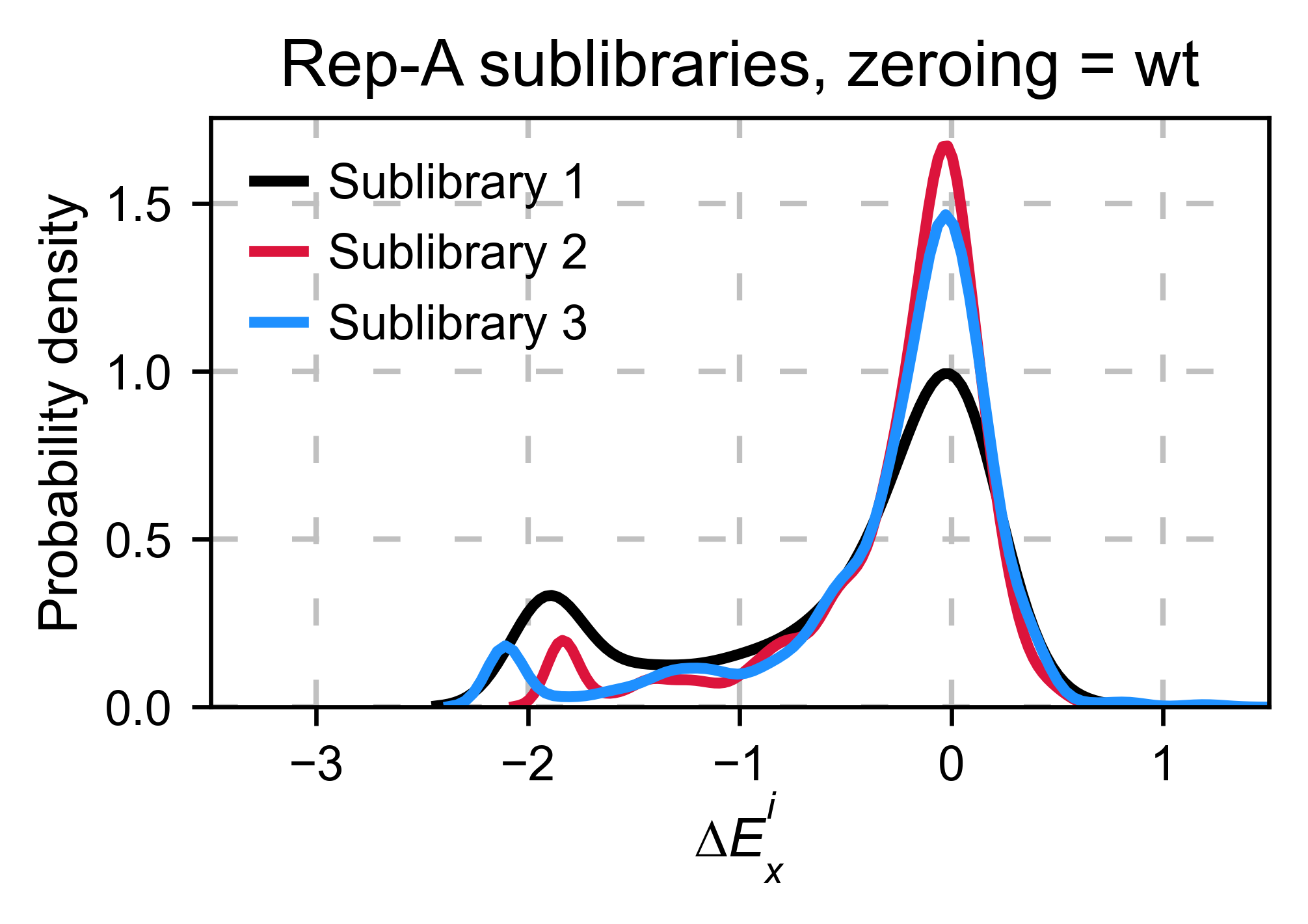## Heatmaps¶

Function and class used in this section:

We are going to evaluate how does the heatmap of produced by each of the normalization methods. We are not going to scale the data, so some heatmaps may look more washed out than others. That is not an issue since can easily be changed by using `std_scale`.

```# First we need to create the objects

# Define protein sequence
hras_sequence: str = 'MTEYKLVVVGAGGVGKSALTIQLIQNHFVDEYDPTIEDSYRKQVVIDGETCLLDILDTAGQEEY'\
+ 'SAMRDQYMRTGEGFLCVFAINNTKSFEDIHQYREQIKRVKDSDDVPMVLVGNKCDLAARTVES'\
+ 'RQAQDLARSYGIPYIETSAKTRQGVEDAFYTLVREIRQHKLRKLNPPDESGPG'

# Order of amino acid substitutions in the hras_enrichment dataset
aminoacids: List[str] = list('ACDEFGHIKLMNPQRSTVWY*')

# First residue of the hras_enrichment dataset. Because 1-Met was not mutated, the dataset starts at residue 2
start_position: int = 2

# Create objects
objects: Dict[str, Screen] = {}
for key, value in df_lib.items():
temp = Screen(value, hras_sequence, aminoacids, start_position)
objects[key] = temp
```

Now that the objects are created and stored in a dictionary, we will use the method `object.heatmap`. You will note that the first heatmap (“population”) looks a bit washed out. If you look at the kernel distribution, the spread is smaller. The “kernel” and “wt” heatmaps look almost identical, while the “counts” heatmap looks all blue. This is caused by the algorithm not being able to center the data properly, and everything seems to be loss of function. That is why it is important to select the method of normalization that works with your data.

```titles: List[str] = ['population', 'counts', 'wt', 'kernel']

# Create objects
for obj, title in zip(objects.values(), titles):
obj.heatmap(title='Normalization by ' + title + ' method')
```